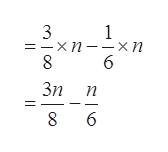Translate into a variable expression. Then simplify.the difference between three-eighths of a number n and one-sixth of the numbervariable expression    simplified expression

Question

Translate into a variable expression. Then simplify.

the difference between three-eighths of a number n and one-sixth of the number
 variable expression simplified expression
Step 1

First, three-eighths is the rational form of 3 and 8. One-sixth is the rational form of 1 and 6.

Step 2

Variable Expression:

The difference between three-eighths of a number n&nb...help_outlineImage Transcriptionclose3 -хп--хп 8 1 6 Зп п б со fullscreen

Want to see the full answer?

See Solution

Want to see this answer and more?

Our solutions are written by experts, many with advanced degrees, and available 24/7

See Solution
Tagged in

Algebra